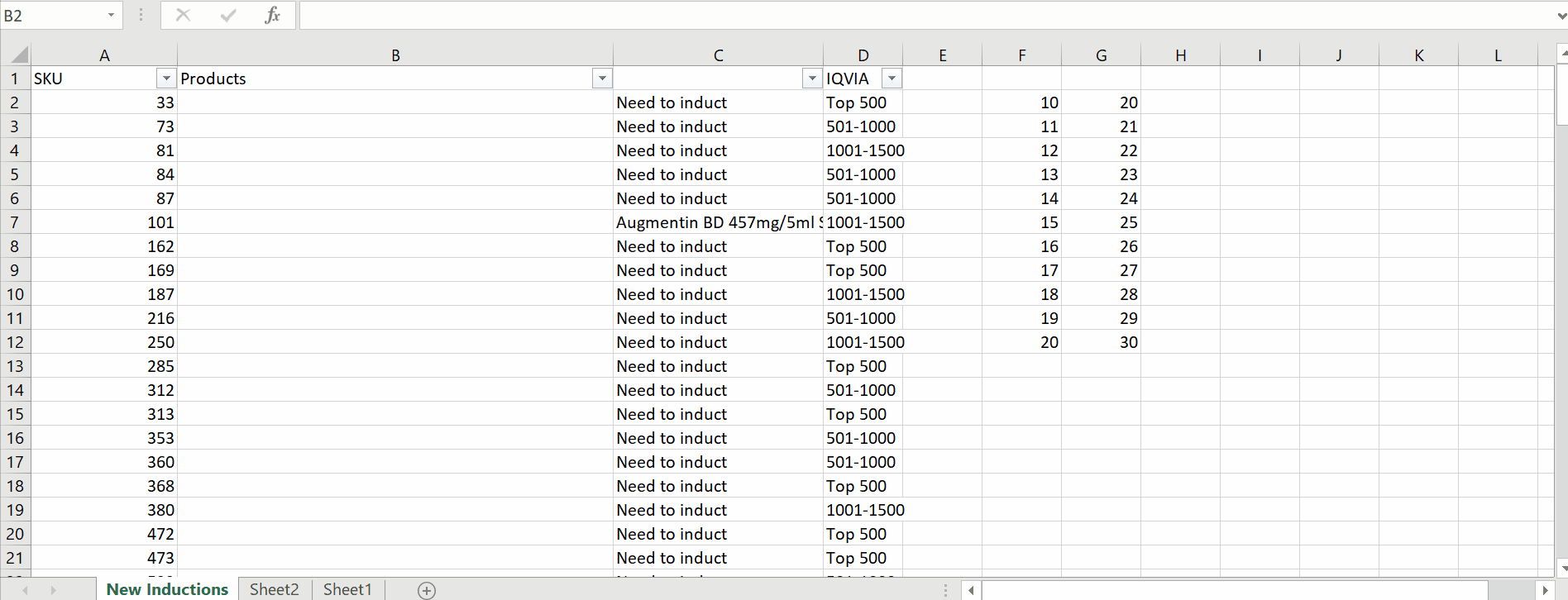# How to pull data from another sheet in Excel

You can watch a video tutorial here.

If you want to pull data from one sheet to another there is a way on the basis of condition. Below is the formula by which we can easily pull our desired values / data range from one sheet to another.

=VLOOKUP (lookup_value, table_array, column_index_num, [range_lookup])

Look up can pick the values from only the first column. It returns the matched value from the table.

lookup_value – it  looks in the first column of a table.

table_array – the table from which to retrieve a value.

column_index_num – The column in the table from which to retrieve a value.

range_lookup – [optional] TRUE = approximate match. FALSE = exact match.

The Vlookup function organizes the data vertically; data must appear in the first column after the table is passed into VLOOKUP.

Below are the steps to follow;Excel provides an ability to use multiple spreadsheets. you can create multiple sheets within the same file. This is a very useful tool and keeps your data concatenated even working on two different sheets. Working on one file, having a part of data on another file , can easily be pulled by using Excel’s built in formula.

### Step 1 – create an appropriate formula– In this case, we have SKU IDs in the sheet New Induction and corresponding product names in another sheet named Sheet1. So we will use VLookup function to pull data from one sheet to another by using the following formula;

=VLOOKUP(A2,’SKU and Product’!A:B,2,0)

– In our formula the first argument A2 refers to the SKU IDs (lookup_value) and the second argument ‘SKU and Product’!A:B refers to the actual data table (table_array) from which we will get our product names. The third argument tells Vlookup to get the value from the 2nd column of the data range and the last argument which is zero (False) tells the formula to search for an exact match.

By using this, we have retrieved data from another sheet. Vlookup can work between two different workbooks. There are some other formulas also available to pull the data from other sheets, which are as follows.

• Lookup
• Hlookup
• Xlookup

These formulas work in more of a similar way as vlookup, i.e. we can pull values from other sheets based on a conditional criteria.